# Shared Sampling for Real-Time Alpha Matting

## Transcription

Shared Sampling for Real-Time Alpha Matting
```EUROGRAPHICS 2010 / T. Akenine-Möller and M. Zwicker
(Guest Editors)
Volume 29 (2010), Number 2
Shared Sampling for Real-Time Alpha Matting
Eduardo S. L. Gastal1 and Manuel M. Oliveira1,2
1 Instituto
2 Camera
de Informática, UFRGS
Culture Group, MIT Media Lab
Abstract
Image matting aims at extracting foreground elements from an image by means of color and opacity (alpha) estimation. While a lot of progress has been made in recent years on improving the accuracy of matting techniques,
one common problem persisted: the low speed of matte computation. We present the first real-time matting technique for natural images and videos. Our technique is based on the observation that, for small neighborhoods,
pixels tend to share similar attributes. Therefore, independently treating each pixel in the unknown regions of a
trimap results in a lot of redundant work. We show how this computation can be significantly and safely reduced by
means of a careful selection of pairs of background and foreground samples. Our technique achieves speedups of
up to two orders of magnitude compared to previous ones, while producing high-quality alpha mattes. The quality
of our results has been verified through an independent benchmark. The speed of our technique enables, for the
first time, real-time alpha matting of videos, and has the potential to enable a new class of exciting applications.
Categories and Subject Descriptors (according to ACM CCS):
Segmentation—Pixel classification
1. Introduction
Extraction and compositing of foreground objects are fundamental image and video editing operations. The process of
digital matting aims at accurately extracting foreground objects from images and videos. For this, matting techniques
need to estimate foreground (F) and background (B) colors
for all pixels belonging to an image I, along with opacity (α)
values. These values are related by the compositing Equation 1, where the observed color C p of pixel p is expressed
as a linear combination of Fp and B p , with interpolation parameter α p :
C p = α p Fp + (1 − α p ) B p .
(1)
For natural images, F and B are not constrained to a particular subset of values. Thus, all variables on the right-hand side
of Equation 1 are unknown, making the problem of computing an alpha matte considerably harder.
Due to the highly ill-posed nature of the matting problem, most existing approaches require additional constraints
in the form of user input, either as trimaps or scribbles.
This user-supplied information identifies pixels for which
the opacity value αi is known to be 1 or 0, i.e., known foreground and known background pixels, respectively. The remaining unconstrained pixels are marked as unknown. The
c 2010 The Author(s)
c 2010 The Eurographics Association and Blackwell Publishing Ltd.
Journal compilation Published by Blackwell Publishing, 9600 Garsington Road, Oxford OX4 2DQ, UK and
350 Main Street, Malden, MA 02148, USA.
I.4.6 [Image Processing and Computer Vision]:
goal of a digital matting algorithm is then to compute the
values of α p , Fp , and B p for all pixels labeled as unknown.
Matting techniques can be classified according to the underlying method used for solving the matte [WC07a], which
can be based on sampling, pixel affinities, or a combination
of the two. Most recent matting algorithms [SRR09,LLW08,
RRG08,WC07b] fall in one of the last two categories, where
local affinities are employed in optimization steps for solving or refining the matte. This usually requires the solution
of large linear systems, whose sizes are directly proportional
to the number of unknown pixels in I and, thus, can become quite big. Furthermore, these optimization procedures
solve for α independently of F and B, thus requiring an additional step for reconstructing F and, if necessary, also B.
Consequently, state-of-the-art techniques take from seconds
to minutes to generate alpha mattes for typical images (with
about 1 Megapixels), making the matte creation process a
tedious task. Long offline computations have also prevented
the use of natural scenes in real-time matting applications,
such as live broadcasting.
We present the first real-time matting technique for natural images and videos. Our approach is based on the key
observation that pixels in a small neighborhood tend to have
highly similar values for (α, F, B) triplets. Thus, a significant
E. S. L. Gastal & M. M. Oliveira / Shared Sampling for Real-Time Alpha Matting
Figure 1: Example of alpha matte extraction and compositing using our technique. (Left) Image (800 × 563 pixels) from the
training dataset provided by [RRW∗ 09]. (Center) Alpha matte computed with our technique in 0.043 seconds using a trimap
with 40% of unknown pixels. (Right) Composite of the extracted foreground on a new background.
amount of computation used to obtain the matte for neighboring pixels is in fact redundant and can be safely eliminated. We show how to avoid such unnecessary computation
by carefully distributing the work among neighboring pixels,
which will then share their results. Since the operations performed by the pixels are independent, they can be performed
in parallel on modern GPUs. As a result, our approach can
generate high-quality mattes up to 100 times faster than the
previous techniques. The quality of our results have been
confirmed by the independent image-matting benchmark of
Rhemann et al. [RRW∗ 09]. According to this benchmark,
our technique ranked second among current state-of-the-art
techniques. Figure 1 shows an example of an alpha matte
extracted with our technique in 0.043 seconds for a challenging example taken from the training dataset provided by
Rhemann et al. [RRW∗ 09]. When extended with an additional optimization step described in Section 4.2, our approach ranks first in the same benchmark, while still performing at interactive rates.
We also introduce a new objective function for identifying good pairs of background and foreground colors for any
given pixel p (Equation 7). Our new function takes into account spatial, photometric, and probabilistic information extracted from the image. Such a function allows our approach
to achieve high-quality results while still operating on a considerably small discrete search space.
Due to its speed, our technique has the potential to enable
new and exciting real-time applications that have not been
previously possible. We illustrate this potential by showing
the first real-time alpha matting demonstration for naturalscene videos, and by providing real-time feedback to users
during interactive alpha-matting extraction sessions.
2. Related Work
Sampling-based approaches make the assumption that the
true foreground and background colors of an unknown pixel
can be explicitly estimated by analyzing nearby known pixels (i.e., pixels in the trimap’s known regions), which are
called background or foreground samples. The first technique to use sampling for estimating the alpha values of
unknown pixels was proposed by Mishima [Mis94]. Earlier
systems also include the work of Ruzon and Tomasi [RT00],
where alpha values are estimated using simple color distributions. Bayesian matting [CCSS01] models the foreground
and background distributions with spatially-varying sets of
Gaussians and solves the matte using a maximum a posteriori (MAP) estimation.
Affinity-based approaches solve for α independent of the
estimation of foreground and background colors. The Poisson matting algorithm [SJTS04] observes that if the foreground and background colors are locally smooth, the gradient of the matte can be estimated from the gradient of the
image. The matte is then found by solving Poisson equations, with boundary conditions defined by the trimap. The
Random Walks method of Grady et al. [GSAW05] propagates user constraints to the entire image by minimizing
a quadratic cost function. The Closed-form matting technique [LLW08] solves the matte by minimizing a cost function derived from careful analysis of the matting problem.
Combined approaches mix an initial sampling step with
α-propagation methods. The recent Robust matting approach of Wang and Cohen [WC07b] uses an initial sampling step to adapt an energy function that is then minimized
using random walks. The authors discuss a way to calculate
the confidence of the collected samples, and the computation only uses high confidence samples. The work of Rhemann et al. [RRG08] improves on this idea by proposing
new weights for the confidence metric. Furthermore, they
improve the search for suitable foreground samples by assuming that the foreground object is spatially connected.
Some algorithms use additional information to constrain
the matting problem. This extra information usually requires
special conditions, such as capturing multiple images with
different focal depths [MMP∗ 05] or acquiring depth information using a time-of-flight sensor [CTP∗ 08].
Interactive alpha matting of images is typically performed
c 2010 The Author(s)
c 2010 The Eurographics Association and Blackwell Publishing Ltd.
Journal compilation E. S. L. Gastal & M. M. Oliveira / Shared Sampling for Real-Time Alpha Matting
using a two-step iterative process: first, the user refines the
needed constraints (trimap or scribbles), which will then be
used for matte generation in a subsequent step. This process
is repeated until the user is satisfied with the quality of the
matte. Any delay between successive evaluations of the first
step are enough to make this a time-consuming and tedious
process. The system proposed by Wang et al. [WAC07]
tries to avoid such a delay by noting that the user modifies the system constraints in a localized fashion. Therefore, the matte only needs to be (re)computed for a small
portion of the image at a time. However, as noted by Rhemann et al. [RRRAS08], long or complex boundaries are
still monotonous and time-consuming to trace.
For segmentation and matting of videos, Bai and Sapiro
[BS07] use the geodesic distance — based on the shortest path on a weighted graph — to interactively make soft
segmentation and matting of images and videos. The recent
work by Bai et al. [BWSS09] uses local classifiers to propagate a user defined segmentation across time. The authors
extend the work in [LLW08] by adding a temporal coherence term to the cost function for generating mattes for offline video sequences. None of these techniques, however,
are suitable for real-time alpha-matting of videos.
3. Real-Time Alpha Matting
Our technique for real-time alpha matting is based on the
fundamental observation that pixels in a small neighborhood often have highly similar values for their true (α, F, B)
triplets. From this, it follows that: (i) the initial collection
of samples gathered by nearby pixels differ only by a small
number of elements; and (ii) close-by pixels usually select
the same or very similar pairs for their best foreground and
background colors. This leads to the conclusion that performing the alpha matte computation for each pixel independently of its neighbors results in a large amount of redundant
work, which can be safely avoided without compromising
the quality of the matte. In fact, as demonstrated in Section 4,
it is possible to achieve speedups of up to two orders of magnitude while still obtaining high-quality results.
Our technique takes as input an image I (or video sequence) and its corresponding trimap(s), and consists of the
following steps:
1. Expansion of Known Regions: extrapolates “known
foreground" and “known background" regions of the
trimap into the “unknown" regions;
2. Sample Selection and Matte Computation: tries to
identify, for each pixel in the unknown regions, the best
pair of foreground and background samples. It also computes an alpha matte from the obtained samples;
3. Local Smoothing: locally smooths the resulting matte
while maintaining its distinct features.
c 2010 The Author(s)
c 2010 The Eurographics Association and Blackwell Publishing Ltd.
Journal compilation 3.1. Expansion of Known Regions
A trimap T segments an input image (or video frame) into
three non-overlapping pixel regions: known foreground (T f ),
known background (Tb ) and unknown (Tu ). The idea behind
expanding known regions is to exploit the affinity of neighboring pixels to reduce the size of the unknown regions.
Thus, let Dimage (p, q) and Dcolor (p, q) be, respectively, the
image-space and color-space distances between two pixels p
and q. The expansion process consists of checking for each
pixel p ∈ Tu if there exists a pixel q ∈ Tr (r = { f , b}) such
that Dimage (p, q) ≤ ki , Dcolor (p, q) ≤ kc , and Dimage (p, q) is
minimal for p. In such a case, pixel p is labeled as belonging
to region Tr based on its affinity to pixel q ∈ Tr . The value of
the parameter ki depends on the unknown region size; thus,
larger images might require larger values of ki . We found
that ki = 10 pixels and kc = 5/256 units (measured as Euclidean distance in the RGB unit cube) produce good results
for typical images. These values were used for all examples
shown in the paper and supplemental materials.
3.2. Sample Selection and Matte Computation
For each remaining pixel p ∈ Tu , our goal is to find an
(α, F, B) triplet that better models p. For this, a sampling
strategy inspired by the work of Wang and Cohen [WC07b]
is used, but it differs from theirs in some fundamental aspects. For any given pixel p ∈ Tu , Wang and Cohen’s idea
is to collect a large number of foreground and background
samples in a neighborhood around p. Such samples are considered candidates for estimating the alpha value of p. Their
assumption is that the true foreground and background colors should be close to the ones of some of the collected
samples. This is a reasonable assumption when the initial
sample set is large. For instance, in their approach, Wang
and Cohen analyze 400 pairs of foreground and background
colors for each pixel p [WC07b]. Unfortunately, the use of
larger sample sets requires a significant amount of computation. The next sections show that this computational cost
can be significantly reduced by exploiting affinities among
neighboring pixels. Furthermore, a new and improved metric for electing the best samples is presented, which takes
into account image parameters that were not considered in
the sample-selection process described in [WC07b].
We minimize the amount of work involved in finding the
best pairs of foreground and background samples for a set of
neighbor pixels by leveraging their high affinity. For this, we
first divide this task among the various pixels in the neighborhood (sample gathering), which then share and refine
their results (sample refinement):
Sample Gathering: in this stage, each pixel p ∈ Tu selects
the best (F, B) pair from a small set of samples in its neighborhood. Those sets are gathered in a manner that guarantees that sample sets from neighboring pixels are disjoint. p
gathers at most kg background and kg foreground samples
E. S. L. Gastal & M. M. Oliveira / Shared Sampling for Real-Time Alpha Matting
Minimization of chromatic distortion: Similar to what has
been described by Wang and Cohen [WC07b], our approach
favors the selection of pairs of foreground and background
colors that can model the color of pixel p as a linear combination of themselves. This is modeled by the chromatic
distortion M p , whose value should be small for a good pair
of candidate colors:
Figure 2: The red arrows starting at p define the paths
for searching for background and foreground samples for p.
The selected (closest) samples are marked in orange. Pixel
q explores a different path (in blue). Foreground samples are
shown as squares, and background samples as circles.
(Figure 2), resulting in at most kg2 tested pairs, from which
the best candidate is selected.
Sample Refinement: in this stage, each pixel p ∈ Tu analyzes the choices (without recombining them) of its (at most)
kr spatially closest pixels in Tu . Thus, while in practice p
performs (at most) kg2 + kr pair evaluations, due to the affinity among neighbor pixels, this is roughly equivalent to performing (at most) kg2 ×kr pair comparisons. According to our
experience, values of kg = 4 and kr = 200 produce very good
results. For these values, the actual number of performed pair
comparisons is 216 (i.e., 16 + 200), while its net effect approximates a total of 3,200 (i.e., 16 × 200) comparisons.
Sections 3.2.1 and 3.2.2 present the details of the sample
gathering and sample refinement sub-steps.
3.2.1. Sample Gathering
In this stage, each pixel p ∈ Tu looks for possible foreground
and background samples along kg line segments starting at
p (Figure 2). These segments divide the plane of the image
into kg disjoint sectors containing equal planar angles. The
slope of the first line segment
associated to p is defined by
an initial orientation θ ∈ 0, π2 measured with respect to the
horizontal line. Such an angle takes a different value for each
pixel q ∈ Tu in a 3×3 window (Figure 2). The orientation of
the other segments is given by an angular increment θinc =
2π
kg . Starting from p and following a particular line segment
yields at most one background and at most one foreground
sample — the ones closer to p along the segment. Thus, p
must find its best pair among, at most, kg2 sample pairs.
We introduce a new objective function that combines photometric, spatial, and probabilistic elements to select the best
sample pair for a pixel from the initial set of kg2 pairs. The
proposed approach is the first to comprehensively consider
all these aspects. Thus, let fi and b j be a pair of foreground
and background samples, whose colors are F i and B j , respectively. Next, we will derive an objective function (Equation 7) for identifying the best sample-pair for each pixel
p ∈ Tu . Before describing this function, its required building blocks (minimization of chromatic distortion and image
space statistics) will be presented.
M p (F i , B j ) = kC p − (α̂ p F i + (1 − α̂ p ) B j )k
(2)
where C p is the color of p, and α̂ p is the estimated alpha value for p, obtained using the color space projection
of C p onto the line defined by F i and B j . Wang and Cohen [WC07b] further divide Equation 2 by kF i − B j k —
which we do not do — to enforce a wide separation of the
foreground from the background colors. While their observation regarding linear interpolation is intuitively sound according to Equation 1, this second criterion is oversimplifying and does not address the fundamental issues involved
in the computation of a matte. As such, it does not represent a good measure for comparison of candidate pairs.
Thus, although a small M p (F i , B j ) is necessary for accurately representing the alpha value of p, it is not a sufficient condition to elect a good sample pair. For this reason,
we propose a new color metric derived from two previously
made observations: (i) Omer and Werman [OW04] showed
that small pixel neighborhoods tend to form locally linear
clusters in color space. This is particularly true for small
windows located over image edges; and (ii) Mitsunaga et
al. [MYT95] showed that if the foreground and background
gradient norms k∇Fk and k∇Bk are relatively small compared to k∇αk, then the image gradient ∇I is directly proportional to ∇α.
Based on these observations, one concludes that in the unknown region of the trimap — where k∇αk is potentially
very large — the locally-linear color variations observed by
Omer and Werman [OW04] are primarily caused by variations in α. Thus, all colors from pixels in a small local window are situated approximately along the line in color space
spanned by the true foreground and background colors F and
B. This means that a good sample pair should minimize the
chromatic distortion not only for p, but for all pixels in a
small window around p. Thus, a good sample pair should
minimize the least squares residual defined by the neighborhood affinity term:
N p (fi , b j ) =
∑
Mq (F i , B j )2
(3)
q∈Ω p
where Ω p is the pixel neighborhood of p, consisting of all
pixels in a 3 × 3 window centered at p, and Mq is the operator defined in Equation 2, evaluated at pixel q.
Image space statistics: In addition to color space information, image space statistics should play a key role in identifying good pair of samples. These image parameters were
not considered in the sample selection process of [WC07b],
where only color space metrics were used. We will now
c 2010 The Author(s)
c 2010 The Eurographics Association and Blackwell Publishing Ltd.
Journal compilation E. S. L. Gastal & M. M. Oliveira / Shared Sampling for Real-Time Alpha Matting
use such information to estimate the probability of pixel p
belonging to the foreground, which will be later used in
Equation 6 to enforce a meaningful alpha value. Thus, let
D p (s) = Dimage (s, p) = ks − pk be the image space distance
from a sample s to the current pixel p. We define the energy
E p (s) to reach a foreground or background sample s from
the current pixel p as the squared path integral of ∇I along
the image space line segment L connecting p and s:
2
Z s
Z
s− p ∇I ·
. (4)
k∇I · drk2 =
E p (s) =
ks − pk p
L
The energy E p (s) is directly proportional to the projected
length of ∇I onto the normalized direction of integration
(s − p). Thus, if the linear path from s to p crosses image
regions where k∇Ik is large (e.g., at image edges), greater
energy will be required to reach s.
An estimate of the probability of p belonging to the foreground, according to the energy E p (s), can be obtained as:
PFp =
min j (E p (b j ))
.
mini (E p (fi )) + min j (E p (b j ))
(5)
Thus, if the minimum energy required to reach a foreground
sample is much lower than the minimum energy required to
reach a background sample, PFp will be close to one — i.e.,
pixel p has a high probability of belonging to the foreground.
Intuitively, we want the alpha value α̂ p (computed from fi
and b j — Equation 2) to correlate with the probability PFp
of pixel p belonging to the foreground. Thus, a good sample
pair should also minimize the function A p :
A p (fi , b j ) = PFp + (1 − 2 PFp ) α̂ p .
(6)
Indeed, for a given pair of samples (fi , b j ), when PFp = 0,
A p (fi , b j ) = α̂ p . Thus, minimizing A p (fi , b j ) also minimizes
the value of α̂ p . Likewise, when PFp = 1, A p (fi , b j ) =
(1 − α̂ p ), so minimizing A p (fi , b j ) maximizes α̂ p . Finally,
if PFp = 0.5, A p (fi , b j ) = 0.5, and the value of α̂ p has no
effect on the minimization. A p (fi , b j ) will be used as one of
the terms in the final objective function (Equation 7).
The Objective function: The resulting objective function
that combines photometric and spatial affinity, as well as
probabilistic information for selecting good pairs of background and foreground samples can be expressed as:
g p (fi , b j ) = N p (fi , b j )eN A p (fi , b j )eA D p (fi )e f D p (bi )eb . (7)
Here, N p (fi , b j ) minimizes chromatic distortion in the 3 × 3
neighborhood around p. A p (fi , b j ) enforces that the computed alpha matte values correlate with the probability of
pixel p belonging to the foreground. D p (fi ) and D p (bi ) enforce the spatial affinity criterion: the background and foreground samples should be as close as possible to p. The constants e{N,A, f ,b} define the penalties for having a high value
in any of these metrics. In practice, we found that values of
eN = 3, eA = 2, e f = 1 and eb = 4 tend to produce good results. Thus, the best pair of foreground and background samples (f̂ p , b̂ p ) for pixel p is obtained by evaluating g p (fi , b j )
c 2010 The Author(s)
c 2010 The Eurographics Association and Blackwell Publishing Ltd.
Journal compilation for all possible sample-pairs:
(f̂ p , b̂ p ) = argmin f,b g p (fi , b j ).
g
(8)
g
Let (Fp , B p ) be the corresponding colors of the best pair
(f̂ p , b̂ p ) for p, obtained in the gathering stage. We then compute σ2f and σ2b as:
σ2f
=
σ2b
=
1
N
1
N
g 2
∑q∈Ω f Cq − Fp ,
g 2
∑q∈Ωb Cq − B p (9)
where Ω f and Ωb are 5×5 pixel neighborhoods centered at
f̂ p and b̂ p , respectively, and N = 25. The values σ2f and σ2b
measure the local color variations in the neighborhoods Ω f
and Ωb assuming small univariate Gaussian color distributions around f̂ p and b̂ p . We will use σ2f and σ2b in the samplerefinement step. Hence, the output of the sample gathering
g
g
g
stage is a tuple τ p = (Fp , B p , σ2f , σ2b ) for each pixel p ∈ Tu .
3.2.2. Sample Refinement
For small values of kg , the number of samples analyzed by
pixel p ∈ Tu during the sample gathering stage is often not
enough to reliably estimate either an alpha value or the true
foreground and background colors. Thus, a more extensive
search is performed by sharing the best results obtained by
all pixels in a neighborhood around p in Tu .
In the sample-refinement stage, each pixel p compares
its own choice of best sample-pair with the choices of its
(at most) kr spatially closest pixels q ∈ Tu . Among those,
g g
the three tuples with the lowest values of M p (Fq , Bq ) are
g
g
g
then averaged to create a new tuple τ̃ p = (F̃p , B̃ p , σ̃2f , σ̃2b )
for p. The purpose of this averaging is to reduce the occurrence of noise in the resulting alpha matte. This procedure
is supported by the observation that neighbor pixels tend
to have similar values of alpha, as well as background and
foreground colors (i.e., neighbor pixels tend to present high
affinity). Therefore, by averaging the best few values in a
given neighborhood, the occurrence of noise is reduced.
The output of the sample-refinement stage for pixel p ∈ Tu
is another tuple τrp = (Fpr , Brp , αrp , f pr ), where:
g 2
Cp
if C p − F̃p ≤ σ̃2f
Fpr =
,
(10)
g
F̃p
otherwise
g 2
Cp
if C p − B̃ p ≤ σ̃2b ,
Brp =
(11)
g
B̃ p
otherwise
(C p − Brp ) · (Fpr − Brp )
,
Fpr − Brp 2
g g exp −λ M p (F̃p , B̃ p )
=
ε
αrp =
f pr
(12)
if Fpr =
6 Brp
. (13)
if Fpr = Brp
Here, the superscript r represents quantities computed in the
sample-refinement stage. The intuition behind the computation of Fpr is that if the color C p of pixel p is sufficiently close
E. S. L. Gastal & M. M. Oliveira / Shared Sampling for Real-Time Alpha Matting
g
to the average color F̃p of the best three foreground samples computed during the gathering stage, then Fpr should be
taken as C p , thus keeping the original color. The case for
Brp is similar. The alpha value αrp is computed as the relative length of the projection of vector (C p − Brp ) onto vector
(Fpr − Brp ), defined by the computed foreground and background colors. Thus, αrp represents the opacity of p. Finally,
f pr expresses the confidence of p in its candidate foreground
and background colors Fpr and Brp . This confidence measure should decrease fast (but not too fast) as the foreground
g
g
and background colors F̃p and B̃ p fail to properly model the
color C p of p. According to our experience, a value of λ = 10
g
produces good results. Additionally, if C p is close to both F̃p
g
and B̃ p , the α value of p cannot be accurately estimated and
the confidence f pr is set to a small value ε = 10−8 .
For completeness, output tuples for pixels outside the unknown region are also defined; thus, for pixels v ∈ T f , we
have τrv = (Fvr = Cv , Brv = Cv , αrv = 1, fvr = 1); and for pixels v ∈ Tb , we have τrv = (Fvr = Cv , Brv = Cv , αrv = 0, fvr = 1).
3.3. Local Smoothing
Although the sample-selection process takes into account
affinities among localized groups of pixels, this is not
enough to prevent discontinuities in the resulting matte.
Thus, an additional step is used to ensure the local smoothness of the final alpha values, while maintaining its distinct features. This is achieved by computing, for each pixel
p ∈ Tu , a weighted average of the tuples τrq of the closest m
neighbors of p in image space (we use a value of m = 100).
Such neighbors can come from either Tu , T f , or Tb . Let Ψ p
be such a neighborhood for pixel p. The weights are defined
in such way that details in the matte are preserved.
The final foreground and background colors Fp and B p of
p are computed as:
(
G Dimage (p, q) fqr αrp − αrq if p 6= q
Wc (p, q) =
,
G Dimage (p, q) fqr
if p = q
h
i
∑q∈Ψ p Wc (p, q) αrq Fqr
i ,
h
(14)
Fp =
∑q∈Ψ p Wc (p, q) αrq
h
i
∑q∈Ψ p Wc (p, q) (1 − αrq ) Brq
h
i
Bp =
(15)
∑q∈Ψ p Wc (p, q) (1 − αrq )
where G is a normalized Gaussian function with variance
σ2 = m/9π pixels. The set Ψ p of the closest m pixels to p
approximates an image space circle with area m = πr2 . The
farthest pixels in Ψ p have a distance of r to p and should
have weights close to zero in the Gaussian (i.e., r = 3 σ).
Thus, m = πr2 = π(3 σ)2 , which solves to σ2 = m/9π.
The weight Wc (p, q) blends the foreground (background)
colors of pixels p and q taking into account: (i) Spatial affinity – the colors of two pixels that are far apart in image
space should not be averaged. This is modeled by the Gaussian function; (ii) Confidence values – pixels with low confidence in their foreground and background samples should
not propagate their uncertainty. (iii) Difference in alpha values – by minimizing k∇Fk and k∇Bk where the estimated
k∇α̂k is large, we make the final ∇α ≈ ∇I; Furthermore,
whenever Cq = Brq , αrq = 0 regardless the value of Fqr ; conversely, whenever Cq = Fqr , αrq = 1 regardless the value of Brq
(Equation 12). Thus, we multiply Fqr by αrq in Equation 14 to
denote that the confidence of pixel q in its foreground color
Fqr is directly proportional to αrq . Similarly, we multiply Brq
by (1 − αrq ) in Equation 15 to denote that the confidence of
pixel q in its background color Brq is inversely proportional
to αrq .
We can now compute the confidence f p of pixel p in
its final foreground and background colors Fp and B p . To
do so, we first define in Equation 17 the mean foregroundbackground distance DFB (in color space) for the neighborhood Ψ p . This mean is weighted by WFB (q) (Equation 16)
which is directly proportional to the confidence fqr of q and
is maximized for values of αrq = 0.5 — where the confidence
of q in both Fqr and Brq is potentially maximal — while being
zero for αq = {0, 1}. DFB will be used next to compute the
final confidence f p .
WFB (q) = fqr αrq 1 − αrq ,
(16)
h
r
i
r
∑q∈Ψ p WFB (q) Fq − Bq
DFB (Ψ p ) =
. (17)
∑q∈Ψ p WFB (q)
The final confidence f p of pixel p in its final foreground
and background colors Fp and B p is modeled by Equation 18. Here, the first term expresses the ratio of the distance
kFp − B p k to the mean foreground-background distance in
the neighborhood Ψ p (clamped to the range [0,1]). This ratio tries to detect pixels whose final foreground and background colors deviate from those in the neighborhood Ψ p .
The second term is analogous to Equation 13 (λ = 10).
kFp − B p k
f p = min 1,
exp − λ M p (Fp , B p ) . (18)
DFB (Ψ p )
Having Fp , B p and f p , we can now compute the final alpha value α p of pixel p. In order to do so, we first define the
low frequency alpha αlp (Equation 20) as the weighted average of alpha values in the neighborhood Ψ p . The weights
Wα (p, q) are proportional to the confidence fqr of q and inversely proportional to the image space distance of p and q.
Additionally, greater weights are given for pixels lying in T f
or Tb (i.e., known pixels).
Wα (p, q) = fqr G Dimage (p, q) + δ(q ∈
/ Tu ), (19)
αlp =
i
h
∑q∈Ψ p Wα (p, q) αrq
∑q∈Ψ p Wα (p, q)
(20)
where δ is a boolean function returning 1 when q ∈
/ Tu ; or 0
c 2010 The Author(s)
c 2010 The Eurographics Association and Blackwell Publishing Ltd.
Journal compilation E. S. L. Gastal & M. M. Oliveira / Shared Sampling for Real-Time Alpha Matting
otherwise. G is the Gaussian function from Wc (Equations 14
and 15).
The final alpha value α p for p is given by Equation 21. This
equation blends the alpha value computed using Fp and B p
with the low frequency alpha αlp , using as blending factor the
final confidence f p . Thus, pixels with a low final confidence
will accept alpha values from higher-confidence neighbors
(modeled by αlp ) to preserve local smoothness.
αp = f p
(C p − B p ) · (Fp − B p )
kFp − B p k2
+ (1 − f p ) αlp . (21)
Finally, the output of the proposed algorithm for the
matting parameters of pixel p ∈ Tu is given by the tuple
(Fp , B p , α p ) with an associated confidence value of f p . For
completeness, the matting parameters for pixels outside the
unknown region are also defined. Thus, for pixels q ∈ T f
we have the tuple (Fq = Cq , Bq = Cq , αq = 1) with confidence fq = 1, and for pixels w ∈ Tb we have the tuple
(Fw = Cw , Bw = Cw , αw = 0) with confidence fw = 1.
4. Results
We have implemented the technique described in the paper
using C++ and GLSL and used it to process a large number of images, and videos. Given that the search space associated with Equation 7 is both small and discrete, its minima is computed by evaluating this equation for its entire
search space and by selecting the sample-pair with the smallest value. Since these operations can be performed independently for each pixel p ∈ Tu , we exploit the inherent parallelism of current GPUs to efficiently perform these searches
in parallel. All the results reported in this paper were obtained on a 2.8 GHz Quad Core PC with 8 GB of memory
and a GeForce GTX 280 with 1 GB of memory.
In order to assess the quality of our results, we used the
benchmark provided by Rhemann et al. [RRW∗ 09]. It evaluates and compares the accuracy of an image-matting technique against the results produced by the state-of-the-art.
Such a benchmark is composed of eight test images publicly
available at www.al phamatting.com, each accompanied by
three trimaps (small, large and user). These images are designed to be challenging representations of natural scenes,
containing examples of highly textured backgrounds, as well
as images where background and foreground colors cannot
be easily differentiated. The ground-truth alpha mattes for
each of the test images are used to assess the quality of the
results, but are not disclosed to the public. As such, Rhemann et al.’s benchmark provides an independent and reliable mechanism for evaluating the quality of digital imagematting algorithms. Tables 2 (a) and (b) show results from
Rhemann et al.’s benchmark and summarize the accuracy
of our technique (Shared Matting - Real-Time) in comparison to others. Our approach ranks second according to the
sum of absolute differences (SAD) and mean squared error
c 2010 The Author(s)
c 2010 The Eurographics Association and Blackwell Publishing Ltd.
Journal compilation Image
elephant
donkey
pineapple
doll
plasticbag
plant
troll
net
# of pixels
536,800
455,200
481,600
451,200
529,600
425,600
512,000
496,000
% Unknown
16%
17%
20%
25%
28%
35%
40%
51%
Time (sec)
0.029
0.028
0.032
0.034
0.040
0.038
0.045
0.056
Table 1: Time to generate alpha mattes with our technique
for the images in the benchmark (Figure 2), using the most
conservative trimaps (i.e., large).
(MSE) metrics, when compared to previous techniques. It is,
however, up to 100 times faster, allowing, for the first time,
alpha matting in real-time applications. Such results indicate
that our technique produce high-quality alpha mattes even
for challenging images.
Since previous technique are not suitable for real-time applications, performance comparisons considering the time
required to compute the alpha matte have been overlook
in many previous publications. Our technique, on the other
hand, can compute alpha mattes for typical images in realtime. Table 1 summarizes the times required to extract the
alpha mattes for the test dataset available from Rhemann
et al.’s benchmark [RRW∗ 09] using the most conservative
trimaps (i.e., large). For each image, we provide its dimensions, the number of pixels in the unknown region of the
trimap, and the time required to compute the matte.
Figure 3 shows the alpha mattes generated by our technique for some images from the training dataset provided
by [RRW∗ 09] (using the small trimaps). For such dataset,
the ground-truth mattes are available and are shown next
to our results for comparison. Versions of these images in
their original resolutions are provided for closer inspections
as supplementary material. For this dataset (average image
size of 0.5Mpix, with 16% unknown pixels), our technique
takes on average 0.024 seconds for the matte computation.
The expansion-of-known-regions step (Section 3.1) reduced
the number of pixels in Tu by 38% on average, but 4% of the
pixels were erroneously considered to be in T f or Tb .
In the gathering stage (Section 3.2.1), foreground and
background samples are found using a linear search (Figure 2) with a step size of 6 pixels and a maximum of 300
steps. Fine details in the trimap might be “jumped over” by
some unknown pixels; however, this is not a problem due to
the sample-pair sharing in the sample-refinement stage.
Cost of the Algorithm: Let z be the number of pixels in
Tu . Per pixel computation can be defined as follows: (i) the
“expansion of known regions” step runs in constant time
O(π ki2 ); (ii) The “sample gathering” step has a worst cost
of O(z + kg2 ), and average cost of O(ω + kg2 ), where ω is the
E. S. L. Gastal & M. M. Oliveira / Shared Sampling for Real-Time Alpha Matting
(a)
(b)
Table 2: Results of Rhemann et al.’s benchmark. Our technique, Shared Matting (Real-Time), ranks second when compared
against previous techniques. However, it is up to 100 times faster. A version of our technique extended with an optimization step
(Shared Matting) ranks first when compared against the same set of techniques. The overal rankings were computed from the
SAD and MSE error values supplied by Rhemann et al.’s benchmark. Only the top five techniques are shown.
Figure 3: Left: Images from the training dataset provided
by [RRW∗ 09]. Center: Alpha mattes extracted with our
technique using the trimaps supplied in the dataset. Right:
Ground truth alpha mattes provided for comparison.
average number of pixels inspected before finding candidate
samples; (iii) the “sample refinement” step runs in constant
time O(kr ); and (iv) the “local smoothing” step runs in constant time O(4 m). All this computation can be done independently for each pixel p ∈ Tu . Thus, let t be the number of
pixels which are processed in parallel on a GPU. The average cost is O(z/t). Worst cost is O(z2 /t).
4.1. Applications
Video Matting Our method enables the use of alpha mat-
ting in real-time applications for the first time. One possible such application is real-time matte generation for videos.
Since our approach uses a trimap as input, it needs to rely
on other techniques for providing trimaps for each frame of
the video in real-time. The supplementary video illustrates
such an application for two video sequences. In these examples, the trimaps were created by dilating the boundaries
of the binary segmented video frames. Such segmentation
was obtained using a real-time foreground-background binary segmentation technique which models the background
color distribution from color priors using the kernel density estimation method (as such, this technique is limited to
videos where the foreground and background color distributions do not overlap). Thus, given an input video sequence,
the results shown in the supplementary video were entirely
computed in real-time. This means that the whole sequence
of operations comprising binary segmentation, boundary dilation, and matte extraction was performed in real time. Figure 4 shows some frames from two of these video sequences
with their corresponding extracted mattes.
Interactive Alpha Matting Another benefit of the improved performance of our technique is its ability to provide
real-time feedback to users during interactive alpha-matting
sessions. We demonstrate this feature using a simple trimap
creation interface. Initially, all pixels in the image are labeled
as belonging to the unknown region Tu . As one uses small
scribbles over the image, a trimap is automatically computed
and refined, also providing instant feedback on the resulting
matte extraction. The scribbles are propagated using an iterative flood-filling procedure, limited by a simple edge detector. Figure 5 illustrates the concept using one of the images
of the training dataset. On the left, one sees the scribbles superimposed onto the original image. The blue color is a label
for foreground pixels, while red and yellow represent backc 2010 The Author(s)
c 2010 The Eurographics Association and Blackwell Publishing Ltd.
Journal compilation E. S. L. Gastal & M. M. Oliveira / Shared Sampling for Real-Time Alpha Matting
4.2. Matte Optimization
Figure 4: Some frames extracted from video sequences processed by our technique for real-time matte extraction. The
images on top show the original frames, while the extracted
mattes are shown at the bottom.
Some users might want to obtain a matte with the best possible quality, even at the expense of extra computation time.
This can be achieved by refining the matte obtained in Section 3 using an additional optimization step. This step is
analogous to the one in [RRG08], where the final matte
is obtained by minimizing a quadratic cost function in α.
This cost function is comprised of a smoothness term and a
data term. Here, we use the matting Laplacian of [LLW08]
as smoothness term, but for the data term we use the matting parameters (α p , f p ) obtained in Section 3. Thus, let
α̂T = [α1 , . . . α p , . . . αn ] be a vector with the alpha values, obtained in Section 3, for all n pixels in the input image.
Let Γ̂ be a diagonal matrix where each diagonal element γ p
is defined as γ p = f p if p ∈ Tu or γ p = 0 if p ∈ T f ∪ Tb . The
final alpha matte is obtained by solving for:
α = argmin αT L α + λ (α − α̂)T D(α − α̂)
+ γ (α − α̂)T Γ̂(α − α̂)
Figure 5: Use of our technique to interactively segment images by alpha matting. As the user scribbles over the image (left), a trimap is automatically updated. The resulting
trimap and alpha matte computed from the set of scribbles
on the left are shown on the center and right images.
ground and unknown pixels, respectively. The image on the
center shows the computed trimap, for which a gray shade
indicates uncertainty about whether a pixel belongs to the
background (shown in black) or to the foreground (shown in
white). The extracted alpha matte is shown on the right.
The speed of our method makes the matte creation process
easier for the user, as there is no delay between input and
matte refinement. This considerably reduces the time taken
to interactively segment images by alpha matting. The supplementary video shows such an application in action. The
resulting mattes are generated considerably faster for images
with complex edges and topologies. Only in the worst case
one needs to completely trace the border of the foreground
object, which is always needed in the technique described
in [WAC07].
High-resolution Matting Due to its improved speed, our
method can generate alpha mattes for high-resolution images
much faster than existing techniques. We ran our algorithm
on the high-resolution training dataset from [RRW∗ 09],
where images are, on average, 6.7 Mpix with 16% unknown
pixels. For these images, matte computation takes a mean
time of 0.3 seconds, with a longest time of 1.1s and shortest time of 0.17s. We also tested our method on extremely
high resolution images. For a 37 Mpix image, for which a
trimap was created manually containing 11% unknown pixels, matte computation took 3.65 seconds (with ki = 30).
c 2010 The Author(s)
c 2010 The Eurographics Association and Blackwell Publishing Ltd.
Journal compilation (22)
where λ is some relatively large number when compared
to to the values in α̂ and Γ̂. γ = 10−1 is a constant which
defines the relative importance of the data and smoothness
terms, L is the matting Laplacian and D is a diagonal matrix
whose diagonal elements are one for pixels in T f ∪ Tb and
zero for all other pixels. Equation 22 yields a sparse linear
system which we solve using the conjugate gradient method
implemented on the GPU. For an image with 512,000 pixels
and 25% unknown pixels, solving for α takes approximately
100ms. Thus, the system is no longer real-time but still interactive. For videos, the matting Laplacian L must be recomputed for every frame. However, such computation for frame
k can be offloaded to the CPU and performed in parallel with
the GPU optimization of frame (k − 1).
This optimization step induces an α-propagation from
high confidence to low confidence pixels. Thus, while pixels
with a high final confidence try to adhere to the alpha values
computed in Section 3, pixels with a low final confidence
will rely more on propagation for their final alpha values.
Tables 2 (a) and (b) show Rhemann et al.’s benchmark
results for the version of our technique containing the additional optimization step (Shared Matting). This extended
version ranks first both in the sum of absolute differences
and mean squared error metrics.
5. Limitations
The proposed technique makes the assumption that the true
foreground and background colors of unknown pixels can
be explicitly estimated by analyzing nearby known pixels.
For images where this assumption does not hold, computed
alpha values will not be accurate (this will be apparent in
the final, low, confidence values). Examples of such problematic images are those containing completely transparent
foreground objects or those where the foreground and background color distributions strongly overlap.
E. S. L. Gastal & M. M. Oliveira / Shared Sampling for Real-Time Alpha Matting
For videos with highly textured backgrounds or greatly
transparent foreground pixels, the produced matte might suffer from temporal noise, i.e. flickering. One can consider
many ways for improving the temporal coherence of the
matte, such as temporal blending of alpha values based on
confidence estimates, or even selecting candidate samples
along the time axis, in addition to the image space.
6. Conclusions and Future Work
We have presented the first real-time matting technique for
natural images and videos. Our technique is based on the observation that pixels in a small neighborhood in image space
tend to have highly similar values for (α, F, B) triplets. As
such, independently computing such triplets for each pixel
in the unknown region in a conventional way tends to result
in a lot of redundant work. The amount of required computation can then be significantly and safely reduced by a careful
selection of pairs of candidate background and foreground
samples. The work required to perform this task can be distributed among neighbor pixels, leading to considerable savings in computation cost. Moreover, the required operations
can be performed in parallel on programmable GPUs.
We have also presented a new objective function for identifying good pairs of background and foreground samples.
Such a function takes into account spatial and photometric, as well as some probabilistic information extracted from
the image. This improved objective function allows our approach to achieve high-quality results while still operating
on a considerably small discrete search space. Our approach
can achieve speedups of up to two orders of magnitude
compared to previous approaches, while producing highlyaccurate alpha mattes. We assessed the quality of our results
by performing the independently-developed benchmark by
Rhemann et al. [RRW∗ 09]. In such a benchmark, our realtime technique ranked second with respect to both the sum of
absolute differences and to the mean squared error metrics.
A version of our technique that uses an additional optimization step to improve the matte quality ranked first according
to both metrics, while still performing at interactive rates.
We have demonstrated that our technique can provide
instant feedback to support interactive extraction of highquality alpha mattes. It is also fast enough to, for the first
time, support alpha-matte computation for natural videos
in real-time, given that the corresponding trimaps are provided. This opens up exciting opportunities for new realtime applications and for improved real-time trimap generation for videos. We are currently exploring some of these
directions. Finally, the problem of handling temporal coherence for real-time matte remains to be explored.
7. Acknowledgements
We would like to thank the anonymous reviewers for
their insightful comments. Manuel M. Oliveira acknowl-
edges the following CNPq-Brazil fellowships and grant:
200284/2009-6, 305613/2007-3, and 476954/2008-8. Eduardo S. L. Gastal acknowledges his PIBIC/CNPq/UFRGS
fellowship.
References
[BS07] BAI X., S APIRO G.: A geodesic framework for fast interactive image and video segmentation and matting. ICCV 1
(2007), 1–8. 3
[BWSS09] BAI X., WANG J., S IMONS D., S APIRO G.: Video
snapcut: robust video object cutout using localized classifiers.
ACM Trans. Graph. 28, 3 (2009), 1–11. 3
[CCSS01] C HUANG Y.-Y., C URLESS B., S ALESIN D. H.,
S ZELISKI R.: A bayesian approach to digital matting. In CVPR
(2001), vol. 2, pp. 264–271. 2
[CTP∗ 08] C RABB R., T RACEY C., P URANIK A., DAVIS J.,
S ANTA C RUZ C., C ANESTA I., S UNNYVALE C.: Real-time
foreground segmentation via range and color imaging. In CVPR
Workshop on Time-of-flight Computer Visionn (2008), pp. 1–5. 2
[GSAW05] G RADY L., S CHIWIETZ T., A HARON S., W ESTER MANN R.: Random walks for interactive alpha-matting. In VIIP
(2005), pp. 423–429. 2
[LLW08] L EVIN A., L ISCHINSKI D., W EISS Y.: A closed-form
solution to natural image matting. TPAMI 30, 2 (2008), 228–242.
1, 2, 3, 9
[Mis94] M ISHIMA Y.: Soft edge chroma-key generation based
upon hexoctahedral color space, Oct. 11 1994. US Patent
5,355,174. 2
[MMP∗ 05] M C G UIRE M., M ATUSIK W., P FISTER H., H UGHES
J. F., D URAND F.: Defocus video matting. ACM Trans. Graph.
24, 3 (2005), 567–576. 2
[MYT95] M ITSUNAGA T., YOKOYAMA T., T OTSUKA T.: Autokey: Human assisted key extraction. In SIGGRAPH (1995),
pp. 265–272. 4
[OW04] O MER I., W ERMAN M.: Color lines: Image specific
color representation. In CVPR (2004), vol. 2, pp. 946–953. 4
[RRG08] R HEMANN C., ROTHER C., G ELAUTZ M.: Improving
color modeling for alpha matting. In BMVC (2008), pp. 1155–
1164. 1, 2, 9
[RRRAS08] R HEMANN C., ROTHER C., R AV-ACHA A., S HARP
T.: High resolution matting via interactive trimap segmentation.
CVPR 1 (2008), 1–8. 3
[RRW∗ 09] R HEMANN C., ROTHER C., WANG J., G ELAUTZ
M., KOHLI P., ROTT P.: A perceptually motivated online benchmark for image matting. In CVPR (2009). 2, 7, 8, 9, 10
[RT00] RUZON M., T OMASI C.: Alpha estimation in natural images. In CVPR (2000), vol. 1, pp. 18–25. 2
[SJTS04] S UN J., J IA J., TANG C.-K., S HUM H.-Y.: Poisson
matting. ACM Trans. Graph. 23, 3 (2004), 315–321. 2
[SRR09] S INGARAJU D., ROTHER C., R HEMANN C.: New appearance models for natural image matting. In CVPR (2009). 1
[WAC07] WANG J., AGRAWALA M., C OHEN M. F.: Soft scissors: an interactive tool for realtime high quality matting. In SIGGRAPH (2007), p. 9. 3, 9
[WC07a] WANG J., C OHEN M. F.: Image and video matting:
a survey. Foundations and Trends in Computer Graphics and
Vision 3, 2 (2007), 97–175. 1
[WC07b] WANG J., C OHEN M. F.: Optimized color sampling for
robust matting. CVPR (2007), 1–8. 1, 2, 3, 4
c 2010 The Author(s)
c 2010 The Eurographics Association and Blackwell Publishing Ltd.
Journal compilation ```

### Press release Tiësto teams with PixMob to redefine Club Life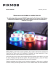More information

### Alpha Industries - A Choice of Heroes (English)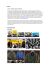More information

### CrowdCompass Graphics Checklist | Cvent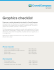More information

### Short Automatic Bar Feeder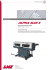More information

### Simultaneous Matting and Compositing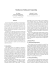More information

### Making a Shepard Fairey Style Poster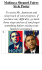More information

### Rasterization: Shading a Triangle Gouraud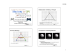More information

### Background Paper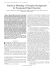More information

### Why Radio?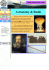More information

### Alpha Epsilon Rho Alpha Kappa Psi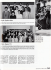More information

### Posters: E-Learning - Electronic Visualization Laboratory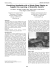More information